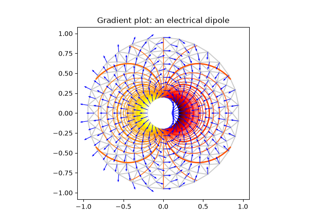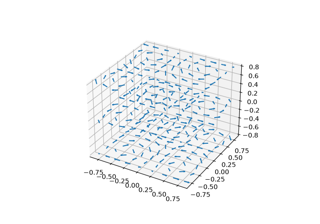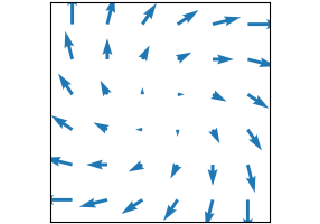# matplotlib.axes.Axes.quiver#

Axes.quiver(*args, data=None, **kwargs)[source]#

Plot a 2D field of arrows.

Call signature:

quiver([X, Y], U, V, [C], **kwargs)


X, Y define the arrow locations, U, V define the arrow directions, and C optionally sets the color.

Arrow length

The default settings auto-scales the length of the arrows to a reasonable size. To change this behavior see the scale and scale_units parameters.

Arrow shape

Arrow styling

Each arrow is internally represented by a filled polygon with a default edge linewidth of 0. As a result, an arrow is rather a filled area, not a line with a head, and PolyCollection properties like linewidth, edgecolor, facecolor, etc. act accordingly.

Parameters:
X, Y1D or 2D array-like, optional

The x and y coordinates of the arrow locations.

If not given, they will be generated as a uniform integer meshgrid based on the dimensions of U and V.

If X and Y are 1D but U, V are 2D, X, Y are expanded to 2D using X, Y = np.meshgrid(X, Y). In this case len(X) and len(Y) must match the column and row dimensions of U and V.

U, V1D or 2D array-like

The x and y direction components of the arrow vectors. The interpretation of these components (in data or in screen space) depends on angles.

U and V must have the same number of elements, matching the number of arrow locations in X, Y. U and V may be masked. Locations masked in any of U, V, and C will not be drawn.

C1D or 2D array-like, optional

Numeric data that defines the arrow colors by colormapping via norm and cmap.

This does not support explicit colors. If you want to set colors directly, use color instead. The size of C must match the number of arrow locations.

angles{'uv', 'xy'} or array-like, default: 'uv'

Method for determining the angle of the arrows.

• 'uv': Arrow direction in screen coordinates. Use this if the arrows symbolize a quantity that is not based on X, Y data coordinates.

If U == V the orientation of the arrow on the plot is 45 degrees counter-clockwise from the horizontal axis (positive to the right).

• 'xy': Arrow direction in data coordinates, i.e. the arrows point from (x, y) to (x+u, y+v). Use this e.g. for plotting a gradient field.

• Arbitrary angles may be specified explicitly as an array of values in degrees, counter-clockwise from the horizontal axis.

In this case U, V is only used to determine the length of the arrows.

Note: inverting a data axis will correspondingly invert the arrows only with angles='xy'.

pivot{'tail', 'mid', 'middle', 'tip'}, default: 'tail'

The part of the arrow that is anchored to the X, Y grid. The arrow rotates about this point.

'mid' is a synonym for 'middle'.

scalefloat, optional

Scales the length of the arrow inversely.

Number of data units per arrow length unit, e.g., m/s per plot width; a smaller scale parameter makes the arrow longer. Default is None.

If None, a simple autoscaling algorithm is used, based on the average vector length and the number of vectors. The arrow length unit is given by the scale_units parameter.

scale_units{'width', 'height', 'dots', 'inches', 'x', 'y', 'xy'}, optional

If the scale kwarg is None, the arrow length unit. Default is None.

e.g. scale_units is 'inches', scale is 2.0, and (u, v) = (1, 0), then the vector will be 0.5 inches long.

If scale_units is 'width' or 'height', then the vector will be half the width/height of the axes.

If scale_units is 'x' then the vector will be 0.5 x-axis units. To plot vectors in the x-y plane, with u and v having the same units as x and y, use angles='xy', scale_units='xy', scale=1.

units{'width', 'height', 'dots', 'inches', 'x', 'y', 'xy'}, default: 'width'

Affects the arrow size (except for the length). In particular, the shaft width is measured in multiples of this unit.

Supported values are:

• 'width', 'height': The width or height of the Axes.

• 'dots', 'inches': Pixels or inches based on the figure dpi.

• 'x', 'y', 'xy': X, Y or $$\sqrt{X^2 + Y^2}$$ in data units.

The following table summarizes how these values affect the visible arrow size under zooming and figure size changes:

units

zoom

figure size change

'x', 'y', 'xy'

arrow size scales

'width', 'height'

arrow size scales

'dots', 'inches'

widthfloat, optional

Shaft width in arrow units. All head parameters are relative to width.

The default depends on choice of units above, and number of vectors; a typical starting value is about 0.005 times the width of the plot.

Head width as multiple of shaft width. See the notes below.

Head length as multiple of shaft width. See the notes below.

Head length at shaft intersection as multiple of shaft width. See the notes below.

minshaftfloat, default: 1

Length below which arrow scales, in units of head length. Do not set this to less than 1, or small arrows will look terrible!

minlengthfloat, default: 1

Minimum length as a multiple of shaft width; if an arrow length is less than this, plot a dot (hexagon) of this diameter instead.

colorcolor or color sequence, optional

Explicit color(s) for the arrows. If C has been set, color has no effect.

This is a synonym for the PolyCollection facecolor parameter.

Returns:
Quiver
Other Parameters:
dataindexable object, optional

If given, all parameters also accept a string s, which is interpreted as data[s] (unless this raises an exception).

**kwargsPolyCollection properties, optional

All other keyword arguments are passed on to PolyCollection:

Property

Description

agg_filter

a filter function, which takes a (m, n, 3) float array and a dpi value, and returns a (m, n, 3) array and two offsets from the bottom left corner of the image

alpha

array-like or scalar or None

animated

bool

antialiased or aa or antialiaseds

bool or list of bools

array

array-like or None

capstyle

CapStyle or {'butt', 'projecting', 'round'}

clim

(vmin: float, vmax: float)

clip_box

Bbox

clip_on

bool

clip_path

Patch or (Path, Transform) or None

cmap

Colormap or str or None

color

color or list of RGBA tuples

edgecolor or ec or edgecolors

color or list of colors or 'face'

facecolor or facecolors or fc

color or list of colors

figure

Figure

gid

str

hatch

{'/', '\', '|', '-', '+', 'x', 'o', 'O', '.', '*'}

in_layout

bool

joinstyle

JoinStyle or {'miter', 'round', 'bevel'}

label

object

linestyle or dashes or linestyles or ls

str or tuple or list thereof

linewidth or linewidths or lw

float or list of floats

mouseover

bool

norm

Normalize or str or None

offset_transform or transOffset

unknown

offsets

(N, 2) or (2,) array-like

path_effects

AbstractPathEffect

paths

list of array-like

picker

None or bool or float or callable

pickradius

unknown

rasterized

bool

sizes

numpy.ndarray or None

sketch_params

(scale: float, length: float, randomness: float)

snap

bool or None

transform

Transform

url

str

urls

list of str or None

verts

list of array-like

verts_and_codes

unknown

visible

bool

zorder

float

Axes.quiverkey

Add a key to a quiver plot.

Notes

Arrow shape

The arrow is drawn as a polygon using the nodes as shown below. The values headwidth, headlength, and headaxislength are in units of width.The defaults give a slightly swept-back arrow. Here are some guidelines how to get other head shapes:

• To make the head smaller relative to the shaft, scale down all the head parameters proportionally.

• To remove the head completely, set all head parameters to 0.

## Examples using matplotlib.axes.Axes.quiver#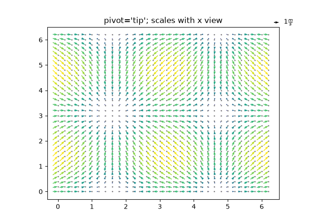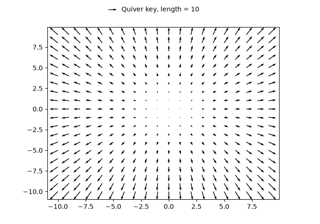Quiver Simple Demo

Quiver Simple Demo